[Home]

#### A Fifth GeneralizationWe derive some relationships of our recurrence formula

A ( n+2 ) = m · A ( n+1 ) - A ( n )

#### 1. ∑ A ( n ) :

We rewrite the equation for A ( n ):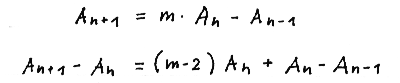We sum up both sides of the equation over n yielding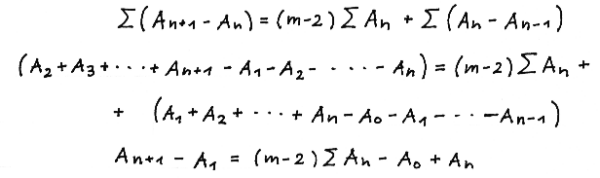Observing A ( 2 ) = m · A ( 1 ) - A ( 0 ) finally yields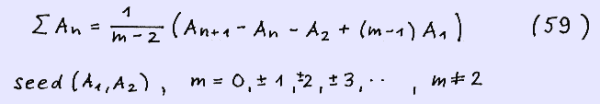The binomial summation formula is achieved using equation (30)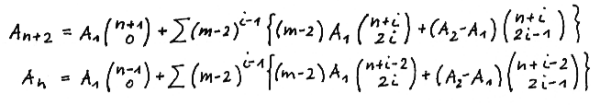Writing equation (30) in full yields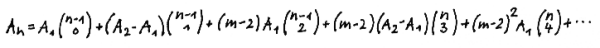Summing up over n yields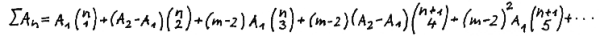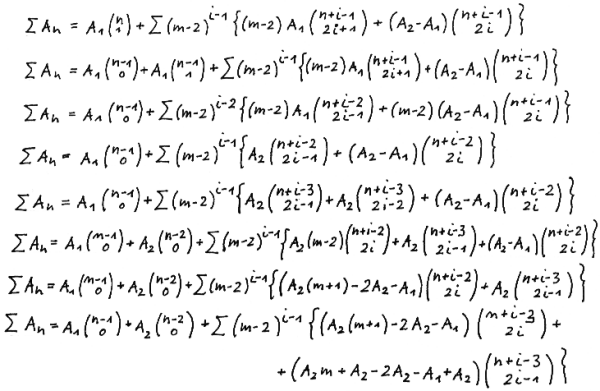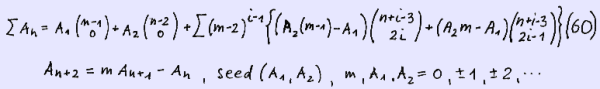We give an example for n = 5:

m = 4 , A (1 ) = 1 , A ( 2 ) = 4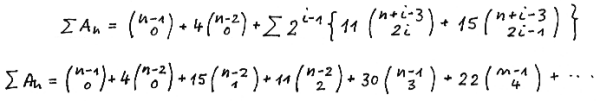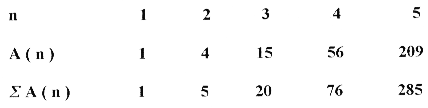We present ∑ A ( n ) in the left - justified manner: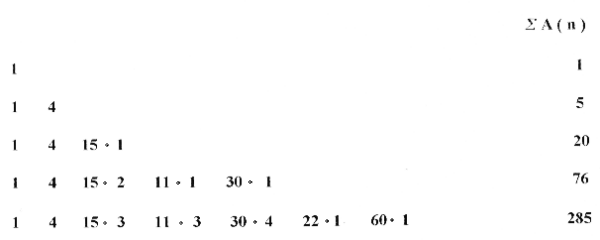We ask now for a recurrence formula for ∑ A ( n ) and give the answer below and prove it later for a more general formula: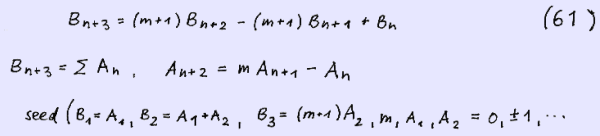#### 2. A ( 2n-1 ) , A ( 2n )

We start from equation A ( n ) = m · A ( n-1 ) - A ( n-2 ).

Observing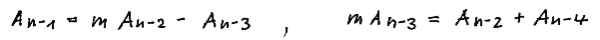yields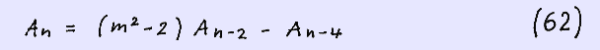So we achieve a correlation between every second element of the sequence which is of the same form as the starting recurrence formula but m replaced by m2 -2 and the seeds replaced in case of A ( 2n-1 ) by the seed ( A ( 1 ) , m · A ( 2 ) - A ( 1 ) ) , in case of A ( 2n ) by the seed ( A ( 2 ) , ( m - 1 ) A ( 2 ) - m A ( 1 ) ) yielding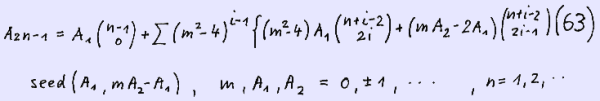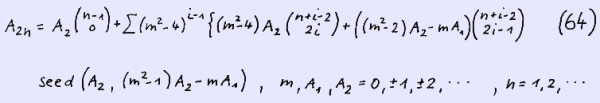#### 3. ∑ A ( 2n-1 ) , ∑ A ( 2n ) :

We sum up both sides of the equations (63) and (64) over n and obtain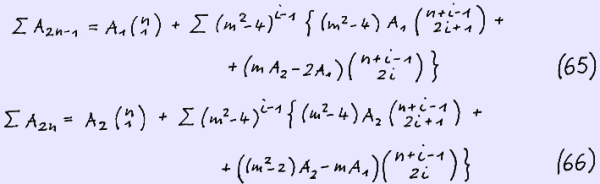We give an example for ∑ A ( 2n ) , m = -3 , seed ( - 2 , 1 ) and n = 5.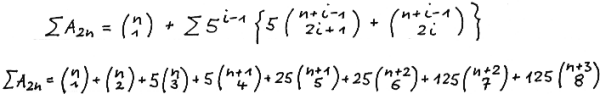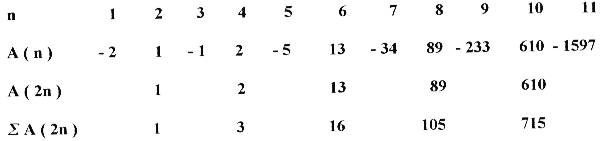We present ∑ A ( 2n ) in the left justified manner.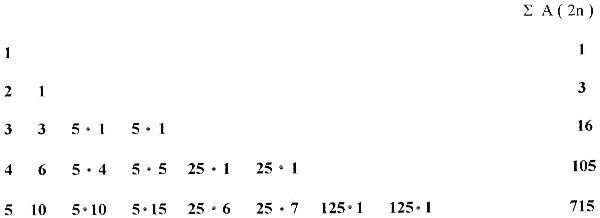We can derive formulae for ∑ A ( 2n ) and ∑ A ( 2n-1 ) equivalent to equation ( 59 ) rewriting equation ( 62).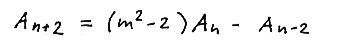Replacing n by 2n and 2n-1 respectively and summing up both sides of the equation over n we obtain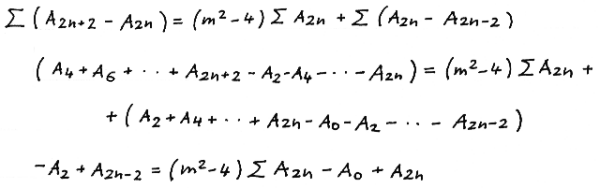Observing A ( 2 ) = m · A ( 1 ) - A ( 0 ) yields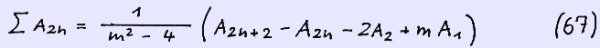In a very similar way we can obtain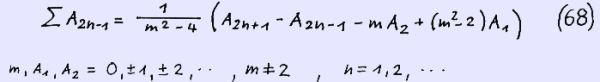In our next page we will present binomial summation formulae for the more general recurrence formula A ( n+2 ) = m · A ( n+1 ) + p · A ( n ) and G ( k n + l ) . You are kindly invited to visit this page.